Question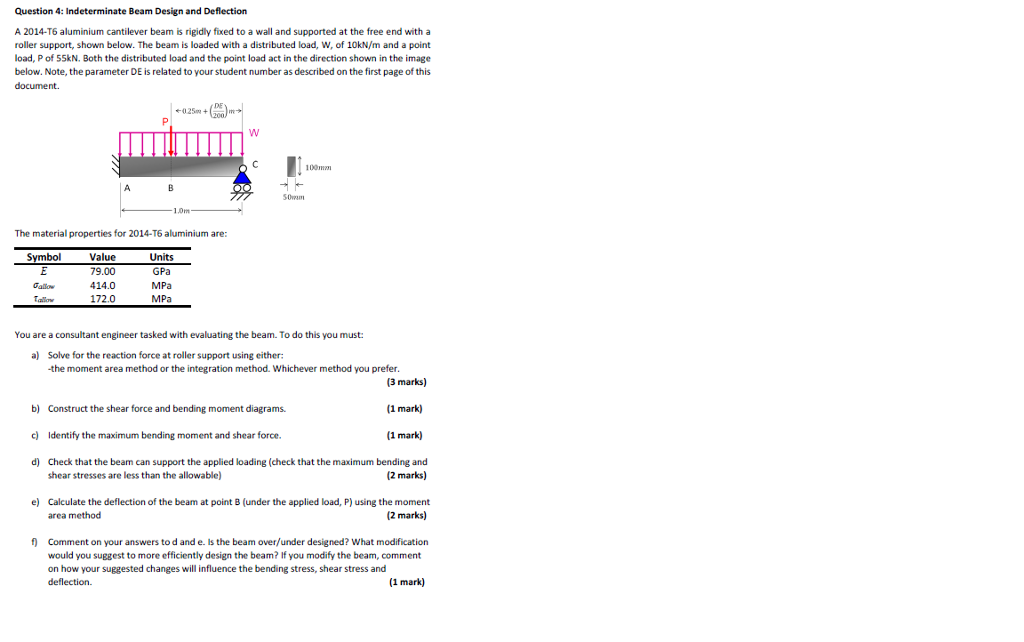DE = 29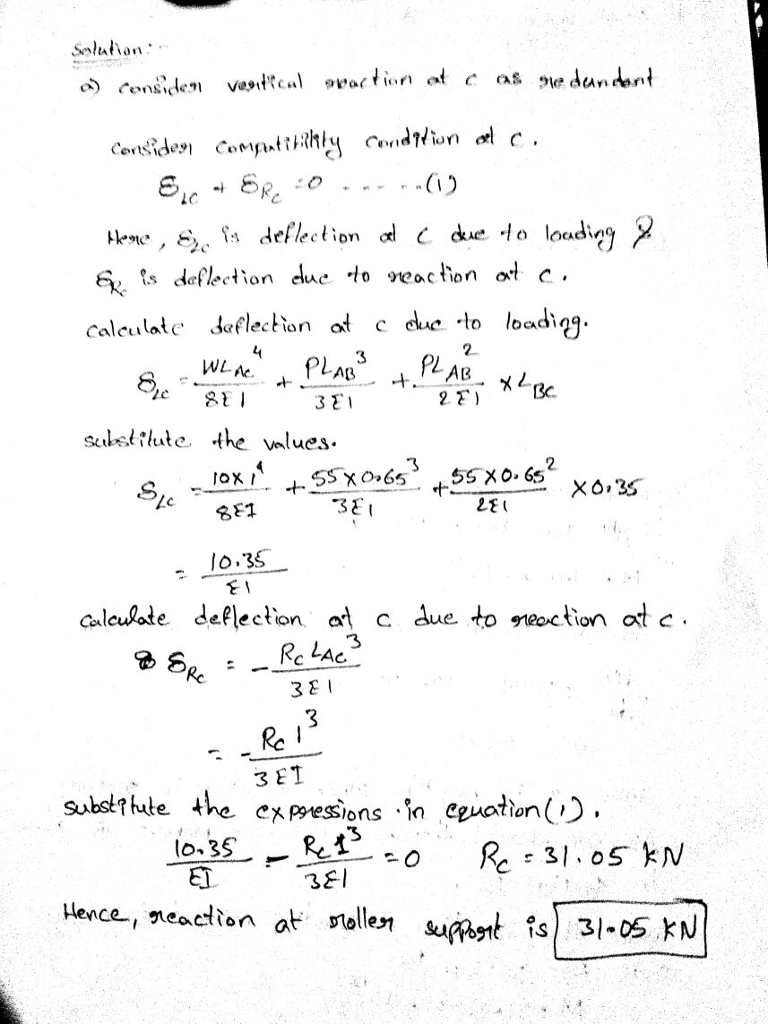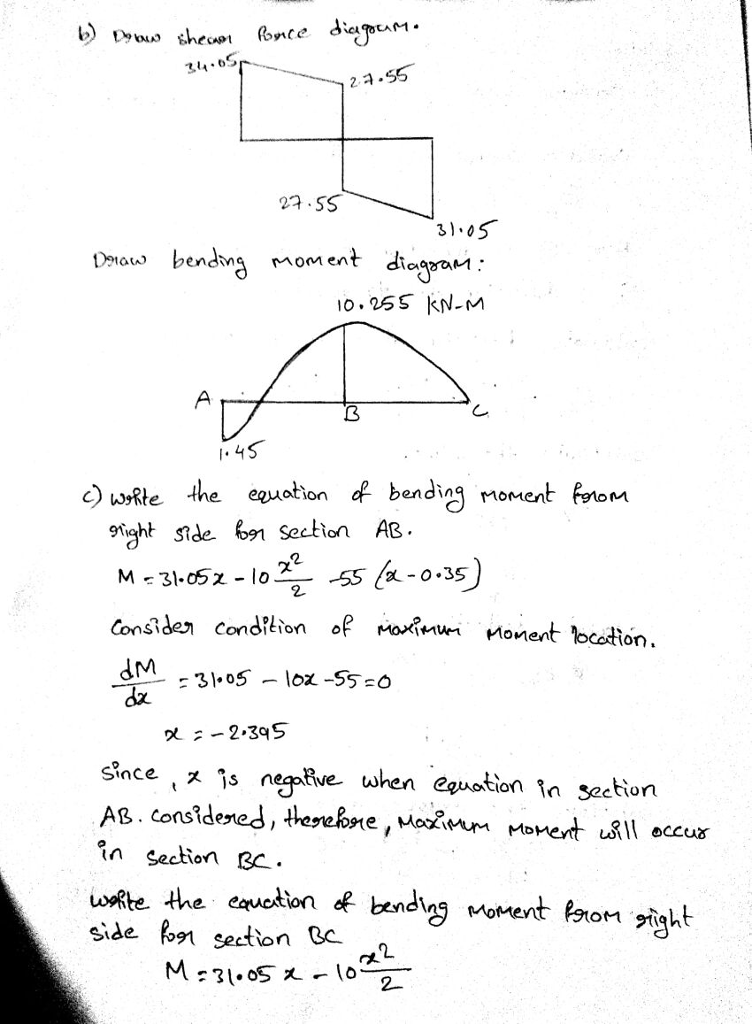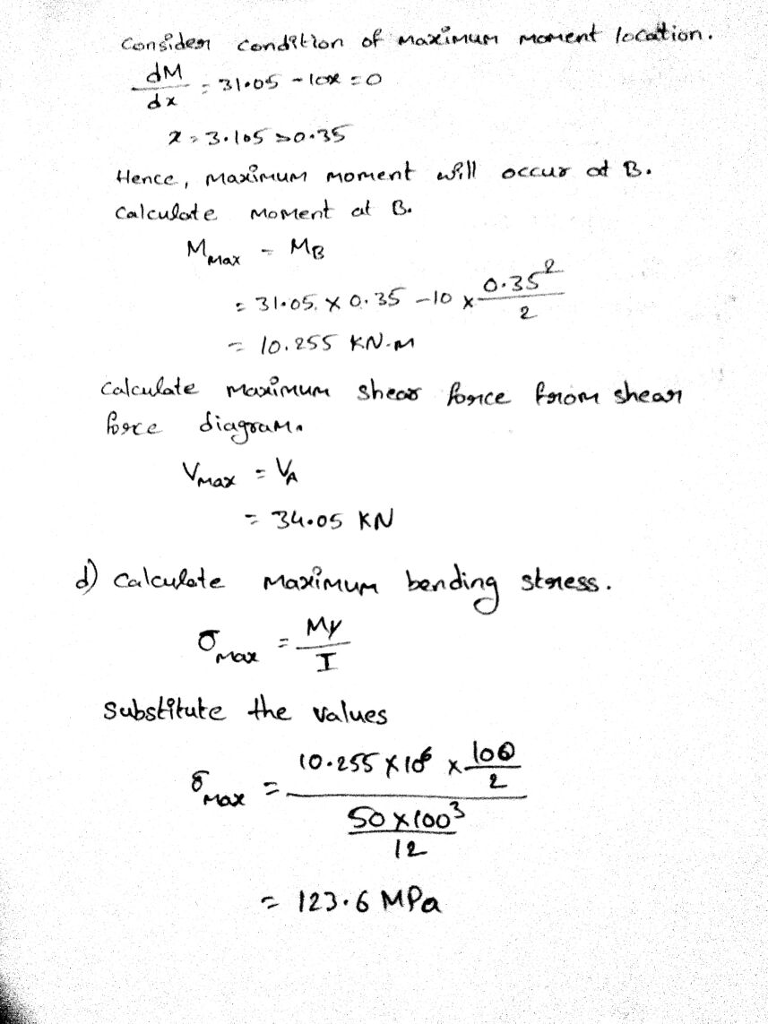#### Earn Coins

Coins can be redeemed for fabulous gifts.

Similar Homework Help Questions
• ### (a) Figure Q3 (a) shows a cantilever beam which is carry a load P at point...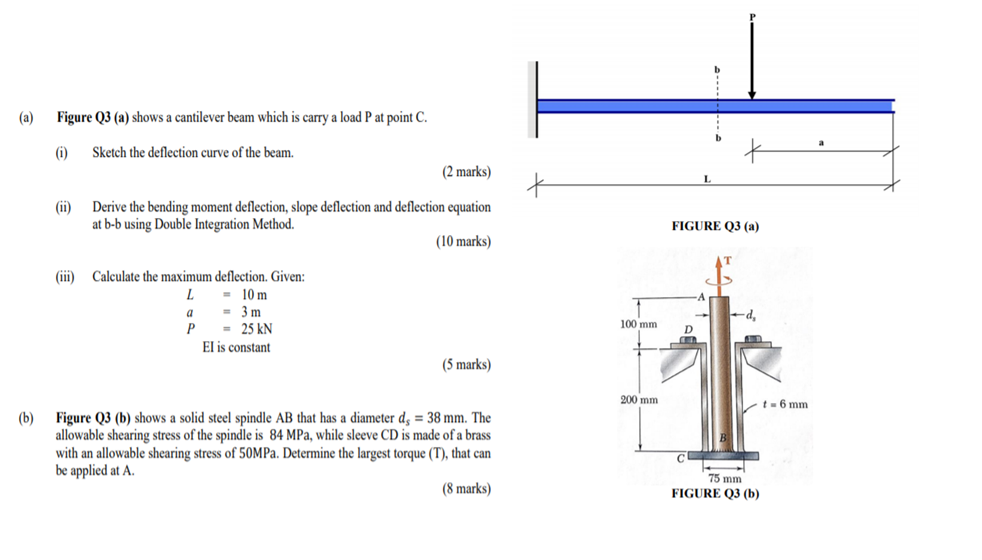(a) Figure Q3 (a) shows a cantilever beam which is carry a load P at point C. (1) Sketch the deflection curve of the beam. (2 marks) t (ii) Derive the bending moment deflection, slope deflection and deflection equation at b-b using Double Integration Method. (10 marks) FIGURE Q3 (a) Calculate the maximum deflection. Given: = 10 m a = 3 m P = 25 KN El is constant d 100 mm D (5 marks) 200 mm t6 mm (b)...

• ### The beam is shown in the figure below. Use the slope-deflection method. The support Ais pinned,...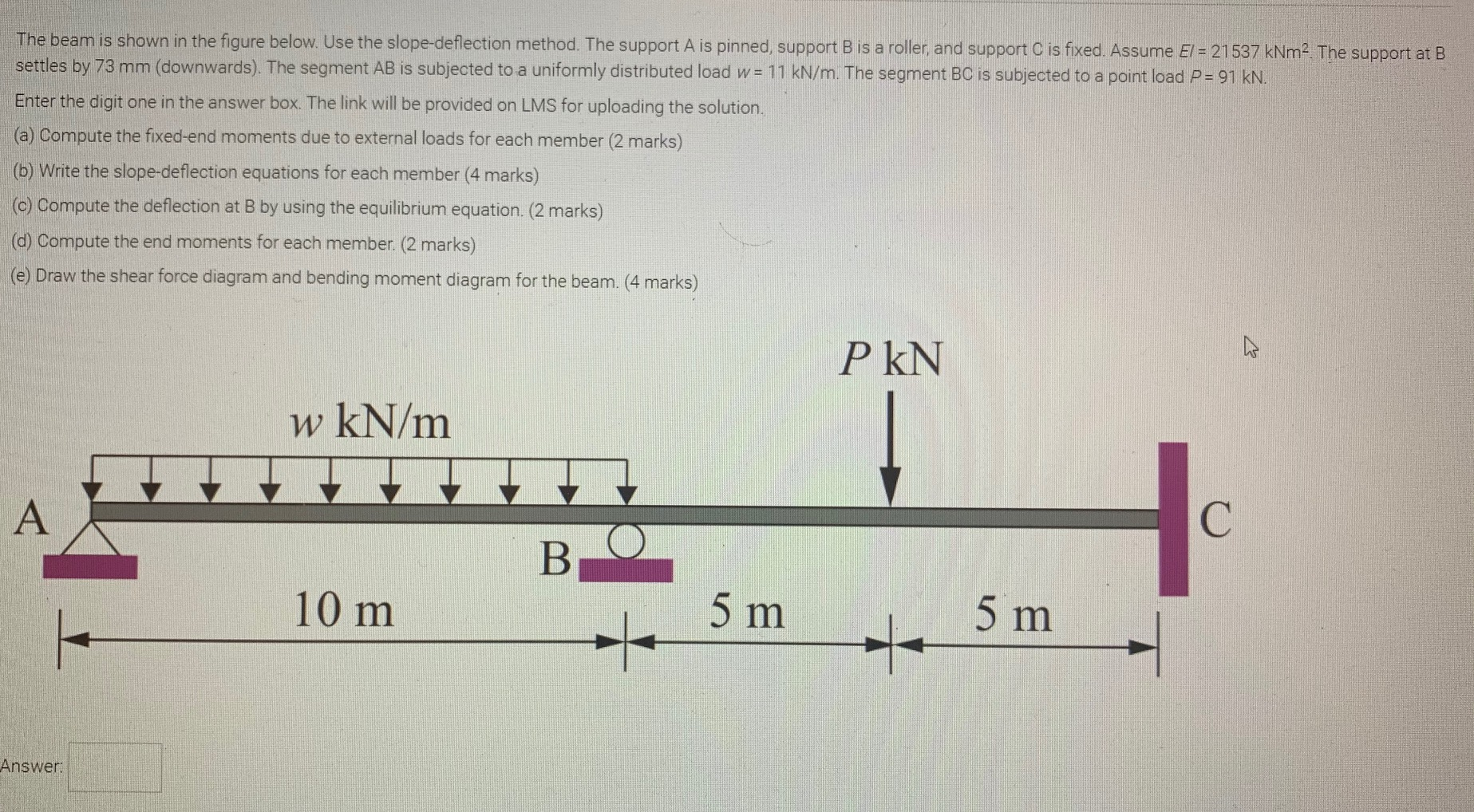The beam is shown in the figure below. Use the slope-deflection method. The support Ais pinned, support B is a roller, and support C is fixed. Assume El = 21537 kNm2. The support at B settles by 73 mm (downwards). The segment AB is subjected to a uniformly distributed load w= 11 kN/m. The segment BC is subjected to a point load P = 91 KN. Enter the digit one in the answer box. The link will be provided on...

• ### Question 1 1. A steel beam is loaded as shown complete the following a. Draw a...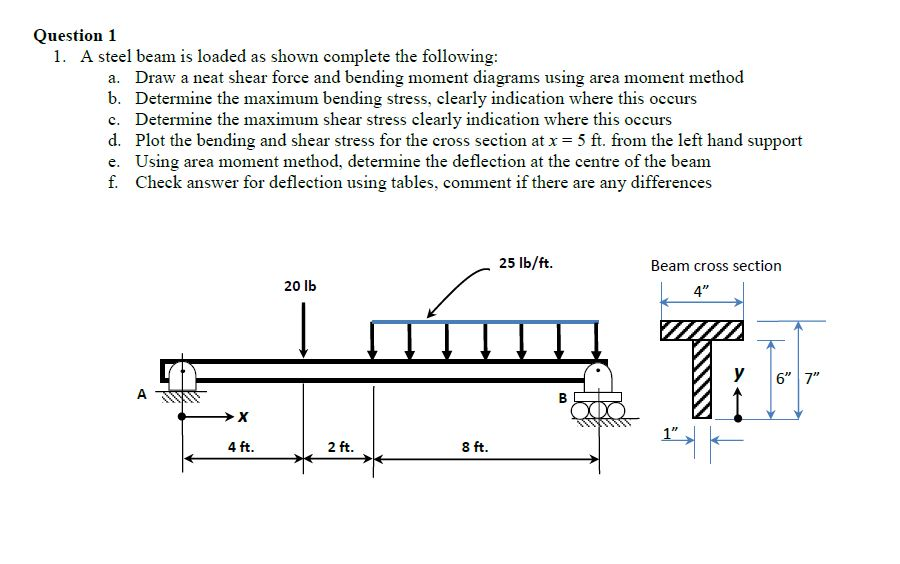Question 1 1. A steel beam is loaded as shown complete the following a. Draw a neat shear force and bending moment diagrams using area moment method b. Determine the maximum bending stress, clearly indication where this occurs c. Determine the maximum shear stress clearly indication where this occurs d, Plot the bending and shear stress for the cross section at x = 5 ft. from the left hand support e. Using area moment method, determine the deflection at the...

• ### A beam may have zero shear stress at a section but may not have zero deflection;...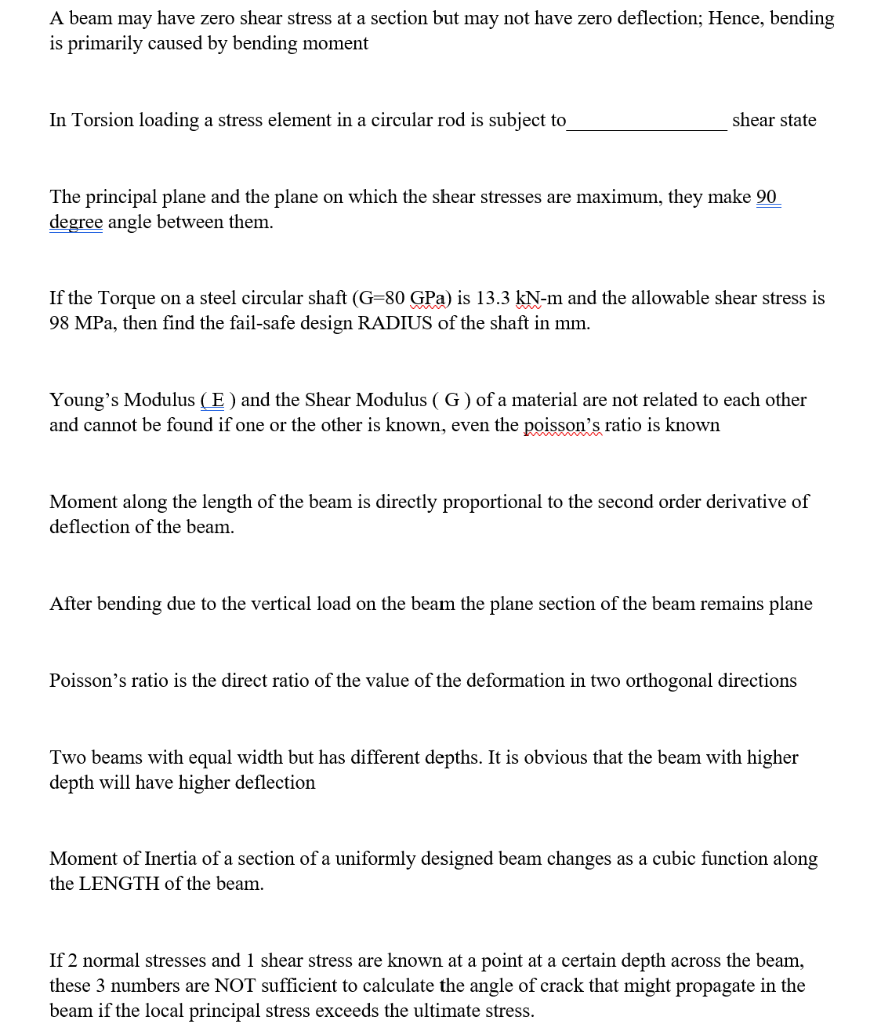A beam may have zero shear stress at a section but may not have zero deflection; Hence, bending is primarily caused by bending moment In Torsion loading a stress element in a circular rod is subject to shear state The principal plane and the plane on which the shear stresses are maximum, they make 90 degree angle between them. If the Torque on a steel circular shaft (G=80 GPa) is 13.3 kN-m and the allowable shear stress is 98 MPa,...

• ### QUESTION 2 Beam ABCD is 8 m in length and is pin-supported at A and roller-supported...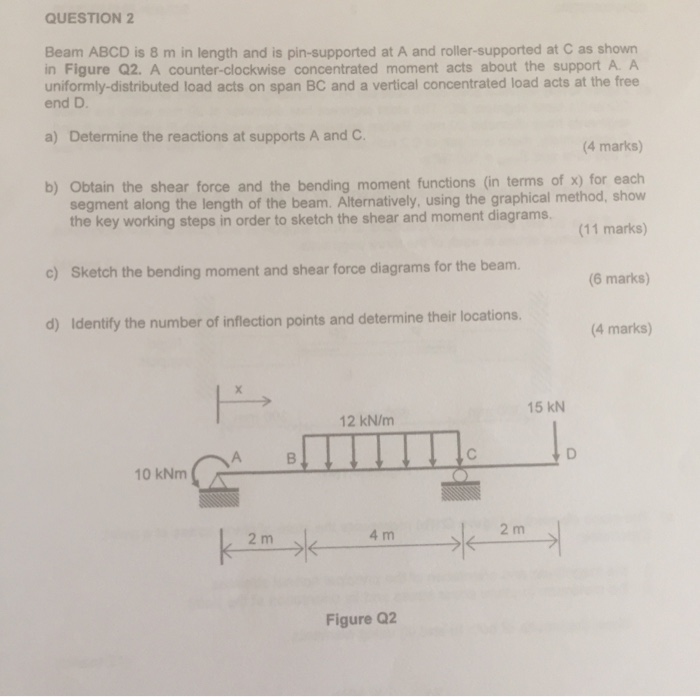QUESTION 2 Beam ABCD is 8 m in length and is pin-supported at A and roller-supported at C as shown in Figure Q2. A counter-clockwise concentrated moment acts about the support A. A uniformly-distributed load acts on span BC and a vertical concentrated load acts at the free end D a) Determine the reactions at supports A and C. 4 marks) b) Obtain the shear force and the bending moment functions (in terms of x) for each segment along the...

• ### Draw the Shear Force (V) and Bending Moment (MI) diagrams of statically indeterminate beam shown in...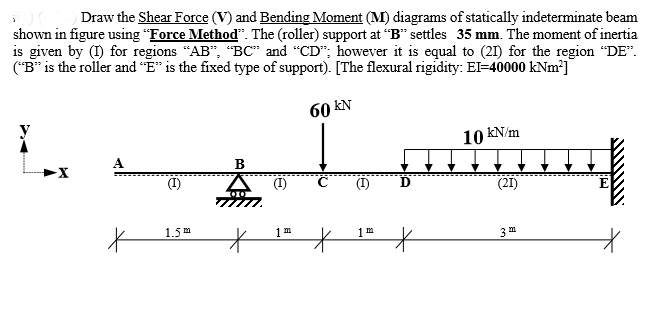Draw the Shear Force (V) and Bending Moment (MI) diagrams of statically indeterminate beam shown in figure using “Force Method”. The (roller) support at "B" settles 35 mm. The moment of inertia is given by (1) for regions "AB", "BC" and "CD"; however it is equal to (21) for the region “DE”. ("B" is the roller and “E" is the fixed type of support). [The flexural rigidity: EI=40000 kNm] 60 KN y 10 kN/m A - Tu (21) 1.5m 11...

• ### #1) (65p.) Draw the Shear Force (V) and Bending Moment (M) diagrams of statically indeterminate beam...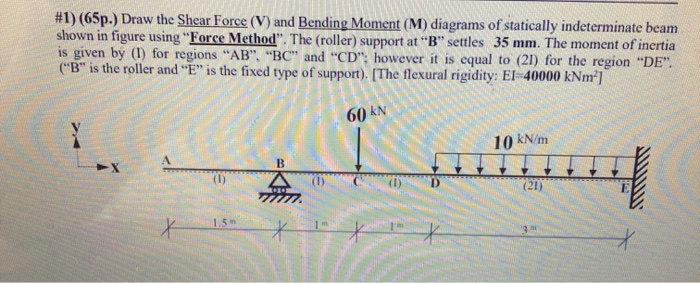#1) (65p.) Draw the Shear Force (V) and Bending Moment (M) diagrams of statically indeterminate beam shown in figure using "Force Method". The (roller) support at "B" settles 35 mm. The moment of inertia is given by (1) for regions "AB", "BC" and "CD": however it is equal to (21) for the region "DE". ("B" is the roller and "E" is the fixed type of support). [The flexural rigidity: EI-40000 kNm] 60 KN 10 kN/m B L (21) 1.5 X...

• ### #1) (65p.) Draw the Shear Force (V) and Bending Moment (M) diagrams of statically indeterminate beam...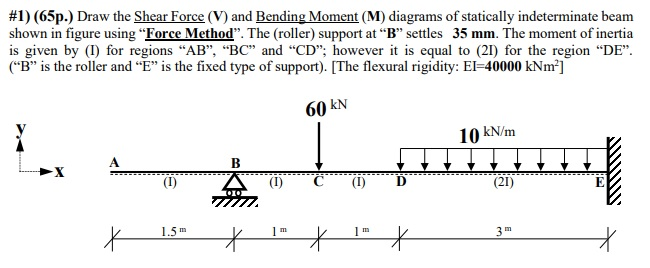#1) (65p.) Draw the Shear Force (V) and Bending Moment (M) diagrams of statically indeterminate beam shown in figure using "Force Method”. The (roller) support at “B” settles 35 mm. The moment of inertia is given by (I) for regions "AB", "BC" and "CD"; however it is equal to (21) for the region “DE”. (“B” is the roller and “E” is the fixed type of support). [The flexural rigidity: EI=40000 kNm] 60 KN 10 kN/m B (21) 1.5 m 1...

• ### #1) (65p.) Draw the Shear Force (V) and Bending Moment (M) diagrams of statically indeterminate beam...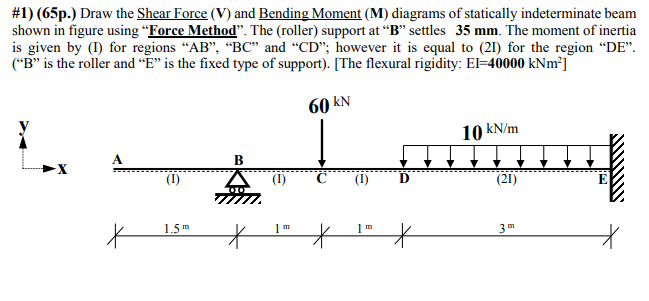#1) (65p.) Draw the Shear Force (V) and Bending Moment (M) diagrams of statically indeterminate beam shown in figure using “Force Method”. The (roller) support at “B” settles 35 mm. The moment of inertia is given by (1) for regions "AB", "BC" and "CD"; however it is equal to (21) for the region “DE”. (“B” is the roller and "E" is the fixed type of support). [The flexural rigidity: EI=40000 kNm-] 60 KN 10 kN/m I. B (21) X 1.5...

• ### #1) (65p.) Draw the Shear Force (V) and Bending Moment (M) diagrams of statically indeterminate beam...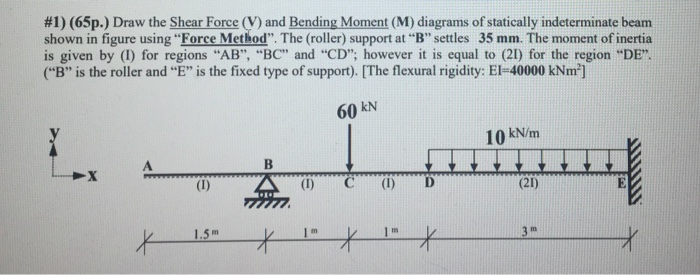#1) (65p.) Draw the Shear Force (V) and Bending Moment (M) diagrams of statically indeterminate beam shown in figure using “Force Method". The (roller) support at "B" settles 35 mm. The moment of inertia is given by (1) for regions "AB", "BC" and "CD"; however it is equal to (21) for the region "DE". ("B" is the roller and "E" is the fixed type of support). [The flexural rigidity: El-40000 kNm"] 60 KN 10 kN/m B (1) (1) D (21)...